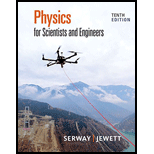Chapter 38, Problem 6P### Physics for Scientists and Enginee...

10th Edition
Raymond A. Serway + 1 other
ISBN: 9781337553278

#### Solutions

Chapter
Section### Physics for Scientists and Enginee...

10th Edition
Raymond A. Serway + 1 other
ISBN: 9781337553278
Textbook Problem

# An astronaut is traveling in a space vehicle moving at 0.500c relative to the Earth. The astronaut measures her pulse rate at 75.0 beats per minute. Signals generated by the astronaut’s pulse are radioed to the Earth when the vehicle is moving in a direction perpendicular to the line that connects the vehicle with an observer on the Earth. (a) What pulse rate does the Earth-based observer measure? (b) What If? What would be the pulse rate if the speed of the space vehicle were increased to 0.990c?

(a)

To determine
The pulse rate as measured by the earth based observer.

Explanation

Given info: The velocity of astronaut is 0.500c . The pulse rate measured by astronaut is 75 beat per minute.

The pulse rate measured by earth observer,

Pulserate=(pulserate)astronaut1v2c2

Substitute 0.500c for v in the above expression

(b)

To determine
The pulse rate as the speed of the vehicle increases to 0.990c .

### Still sussing out bartleby?

Check out a sample textbook solution.

See a sample solution

#### The Solution to Your Study Problems

Bartleby provides explanations to thousands of textbook problems written by our experts, many with advanced degrees!

Get Started

## Additional Science Solutions

#### Find more solutions based on key concepts